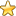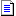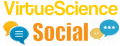Esoteric Wisdom Here are my articles on the Kabbalah, Sacred Geometry and other esoteric subjects. This section needs work and may seem unclear in its present state. Those with a deep curiosity about universal matters should persevere here because there are some very interesting ideas, some of which I have not seen anywhere else.
You are here: HomeEsoteric Wisdom# Pascal's Triangle and CombinatoricsPascal's Triangle can be used to easily work out the number of permutations for a given number of "ingredients" and "places". For example the above diagram highlights that the number of permutations for 3 ingredients over 3 places equals 27:
 AAA AAB ABA BAA AAC ACA CAA ABC ACB BBB BBA BAB ABB BBC BCB CBB BCA BAC CCC CCA CAC ACC CCB CCB BCC CAB CBA

One simply follows the diagonals down from each side. The circle where they meet shows the answer. The smaller number in the circle indicates the number of different Ratios possible for the ingredients and places. In this case it is 10:

 ABC AAA BBB CCC AAB BBA CCA AAC BBC CCB

Note that the small black "Ratio" numbers are identical with the numbers of Pascal's Triangle.

The larger blue "Permutation" numbers follow a simple pattern. The diagonal line from a particular Ingredient Number contains all the powers of that number from 1 to as far as the triangle is extended. For example the 2 Ingredient Line contains all the powers of 2 ie: 1,2,4,8,16,32...etc and the 3 Ingredient Line contains all the powers of 3 ie: 1, 3, 9, 27, 81...etc.

The diagnal line from a particular Place Number contains all the numbers of a particular power from 1 to as far as the triangle is extended. For example the 2 Place Line includes all the Square Numbers ie: 1, 4, 9, 16, 25...etc and the 3 Place Line contains all the cube numbers ie: 1, 8, 27, 64...etc.

You are here: HomeEsoteric WisdomCheck out the latest Esoteric Wisdom Forum Topics: